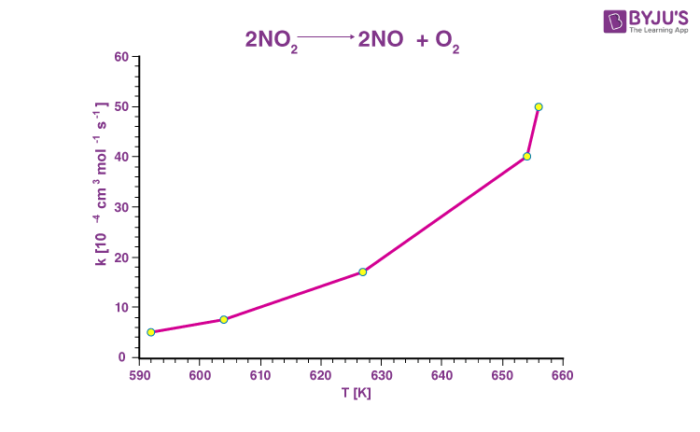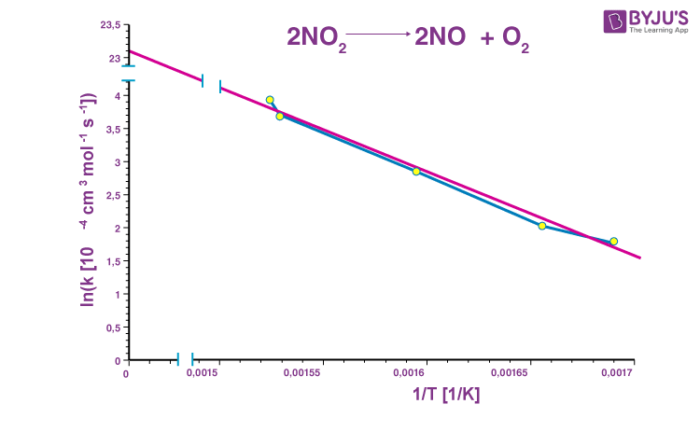Defend yourself better by mastering the science of immunity and vaccines. Secure your free spot, now! Defend yourself better by mastering the science of immunity and vaccines. Secure your free spot, now!

# Arrhenius Equation

The Arrhenius equation is an expression that provides a relationship between the rate constant (of a chemical reaction), the absolute temperature, and the A factor (also known as the pre-exponential factor; can be visualized as the frequency of correctly oriented collisions between reactant particles). It provides insight into the dependence of reaction rates on the absolute temperature.

## What is the Arrhenius Equation?

The expression of the Arrhenius equation is:Where,

• k denotes the rate constant of the reaction
• A denotes the pre-exponential factor which, in terms of the collision theory, is the frequency of correctly oriented collisions between the reacting species
• e is the base of the natural logarithm (Euler’s number)
• Ea denotes the activation energy of the chemical reaction (in terms of energy per mole)
• R denotes the universal gas constant
• T denotes the absolute temperature associated with the reaction (in Kelvin)

If the activation energy is expressed in terms of energy per reactant molecule, the universal gas constant must be replaced with the Boltzmann constant (kB) in the Arrhenius equation. The Arrhenius equation was put forward by the Swedish chemist Svante Arrhenius in the year 1889.

## Graphical Representation of the Arrhenius Equation

For the decomposition reaction undergone by nitrogen dioxide (given by 2NO2 → 2NO + O2), a graph plotted with the rate constant (k) on the Y-axis and the absolute temperature (T) on the X-axis is provided below. Note that the rate of the reaction increases as the temperature increases.### Arrhenius Plot

When logarithms are taken on both sides of the equation, the Arrhenius equation can be written as follows:

ln k = ln(Ae-Ea/RT)

Solving the equation further:

ln k = ln(A) + ln(e-Ea/RT)

ln k = ln(A) + (-Ea/RT) = ln(A) – (Ea/R)(1/T)

Since ln(A) is a constant, the equation corresponds to that of a straight line (y = mx + c) whose slope (m) is -Ea/R. When the logarithm of the rate constant (ln K) is plotted on the Y-axis and the inverse of the absolute temperature (1/T) is plotted on the X-axis, the resulting graph is called an Arrhenius plot.The Arrhenius plot for the decomposition of nitrogen dioxide is illustrated above.

## Does the Arrhenius Equation Account for Catalysts?

The function of a catalyst is to lower the activation energy required by a reaction. Therefore, the lowered activation energy (accounted by the catalyst) can be substituted into the Arrhenius equation in order to obtain the rate constant for the catalysed reaction.

The exponential part of the Arrhenius equation (-Ea/RT) accounts for an exponential increase in the value of the rate constant for any decrease in the activation energy. Since the rate of a chemical reaction is directly proportional to the rate constant of that reaction, the decrease in activation energy results in an exponential increase in the reaction rate.

It is important to note that the rates of uncatalysed reactions are more severely affected by temperature than the rates of catalysed reactions. This is because the activation energy is in the numerator of the exponential term -Ea/RT and the absolute temperature is in the denominator. Since the activation energy of the catalysed reaction is relatively low, the effect of temperature on the rate constant is more visible in the corresponding uncatalysed reaction.

## Arrhenius Equation and the Pre-Exponential Factor (A)

The symbol ‘A’ in the Arrhenius equation denotes the pre-exponential factor or the frequency factor. This factor deals with the collisions between molecules and can be thought of as the frequency of correctly oriented collisions between molecules that have sufficient energy to spark a chemical reaction.

The pre-exponential factor is often represented by the following equation:

A = ⍴Z

Where Z is the frequency factor (frequency of collisions) and ⍴ is the steric factor (deals with orientation of molecules).

The value of A must be determined experimentally since it assumes different values for different reactions. It is also dependent on the temperature at which the reaction is taking place. The units of A are dependent on the order of the reaction. For example, the value of ‘A’ for a second-order rate constant is expressed in L.mol-1.s-1 (or M-1s-1, since M = mol.L-1) and that of a first-order rate constant is expressed in s-1.

### Eliminating the A Factor from the Arrhenius Equation

Considering a chemical reaction at two different temperatures T1 and T2, whose corresponding rate constants are k1 and k2 respectively, the logarithmic form of the Arrhenius equation is:

ln k1 = ln(A) – Ea/RT1

ln k2 = ln(A) – Ea/RT2

The second equation can be rearranged to get the value of ln(A):

ln (A) = ln(k2) + Ea/RT2

Substituting the value of ln(A) in the equation for ln(k1), the following equations can be obtained:

ln(k1) = ln(k2) + Ea/RT2 – Ea/RT1

Shifting ln(k2) to the LHS, the value of ln(k1) – ln(k2) becomes:

ln(k1) – ln(k2) = Ea/RT2 – Ea/RT1

The LHS of the equation is of the form ln(x) – ln(y), which can be simplified to ln(x/y). Also, the term ‘Ea/R’ is a common factor to both the terms in the RHS. Therefore, the entire equation can be simplified as follows:

$$\begin{array}{l}ln(\frac{k_{1}}{k_{2}}) = \frac{-E_{a}}{R}(\frac{1}{T_1} – \frac{1}{T_2})\end{array}$$

## Solved Exercises

### Example 1

The activation energy of a chemical reaction is 100 kJ/mol and it’s A factor is 10 M-1s-1. Find the rate constant of this equation at a temperature of 300 K.

Given,

Ea = 100 kJ.mol-1 = 100000 J.mol-1

A = 10 M-1s-1, ln(A) = 2.3 (approx.)

T = 300 K

The value of the rate constant can be obtained from the logarithmic form of the Arrhenius equation, which is:
ln k = ln(A) – (Ea/RT)

ln k = 2.3 – (100000 J.mol-1)/(8.314 J.mol-1.K-1)*(300K)

ln k = 2.3 – 40.1

ln k = -37.8

k = 3.8341*10-17 M-1s-1 (from the units of the A factor, it can be understood that the reaction is a second-order reaction, for which the unit of k is M-1s-1)

Therefore, the value of the rate constant for the reaction at a temperature of 300K is approximately 3.8341*10-17 M-1s-1.

### Example 2

At a temperature of 600 K, the rate constant of a chemical reaction is 2.75*10-8 M-1s-1. When the temperature is increased to 800K, the rate constant for the same reaction is 1.95*10-7M-1s-1. What is the activation energy of this reaction?

Given,

T1 = 600K

k1 = 2.75*10-8 M-1s-1.

T2 = 800K

K2 = 1.95*10-7M-1s-1

When the A factor is eliminated from the Arrhenius equation, the following equation is obtained:
ln(k1/k2) = (-Ea/R)(1/T1 – 1/T2)

Substituting the given values in the equation, the value of Ea can be determined:

ln(2.75*10-8/1.95*10-7) = (-Ea/8.314 J.K-1.mol-1)*(0.00041K-1)

ln(0.141) = (Ea)*(-0.0000493) J-1.mol

Ea = (-1.958)/(-0.0000493)J.mol-1 = 39716 J.mol-1

The activation energy of the reaction is approximately 39716 J.mol-1.

## Frequently Asked Questions – FAQs

Q1

### What is Arrhenius equation explain with example?

The Arrhenius equation is sometimes expressed as k = Ae-E/RT where k is the rate of chemical reaction, A is a constant depending on the chemicals involved, E is the activation energy, R is the universal gas constant, and T is the temperature.

Q2

### Which theory is the basis for Arrhenius equation?

The number of effective collisions is proportional to the number of molecules above certain threshold energy. As the temperature increases, so does the number of molecules with energies exceeding the threshold energy.

Q3

### What is the effect of catalyst on the rate of reaction?

A catalyst decreases the activation energy barrier of the reaction, thereby increasing the rate of the reaction.

Q4

### What is the value of A and Ea in Arrhenius equation?

In the Arrhenius equation for a certain reaction, the value of A and Ea (energy of activation) are 4×10−13sec−1 and 98. 6 kJ mol−1 respectively.

Q5

### What is true about the Arrhenius equation?

The exponential term in the Arrhenius equation implies that the rate constant of a reaction increases exponentially when the activation energy decreases. Because the rate of a reaction is directly proportional to the rate constant of a reaction, the rate increases exponentially as well.